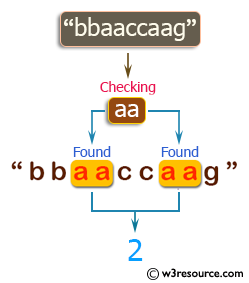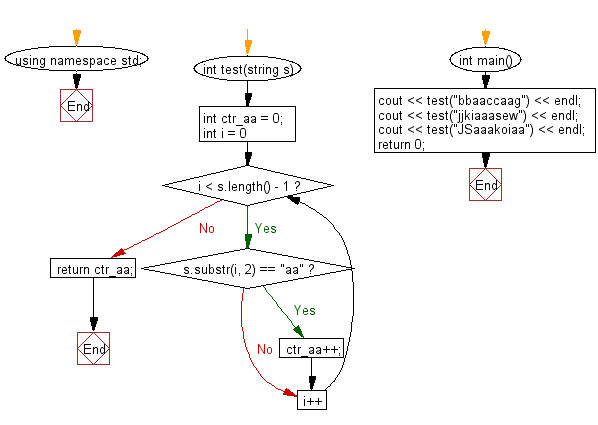﻿ C++ Exercises: Count the string 'aa' in a given string and assume 'aaa' contains two 'aa' - w3resource# C++ Exercises: Count the string 'aa' in a given string and assume 'aaa' contains two 'aa'

## C++ Basic Algorithm: Exercise-26 with Solution

Write a C++ program to count the string "aa" in a given string and assume "aaa" contains two "aa".

Sample Solution:

C++ Code :

``````#include <iostream>
using namespace std;

int test(string s)
{
int ctr_aa = 0;
for (int i = 0; i < s.length() - 1; i++)
{
if (s.substr(i, 2) == "aa")
{
ctr_aa++;
}
}
return ctr_aa;
}

int main()
{
cout << test("bbaaccaag") << endl;
cout << test("jjkiaaasew") << endl;
cout << test("JSaaakoiaa") << endl;
return 0;
}
``````

Sample Output:

```2
2
3
```

Pictorial Presentation:Flowchart:C++ Code Editor:

Contribute your code and comments through Disqus.

What is the difficulty level of this exercise?

﻿